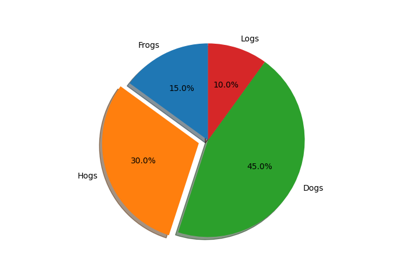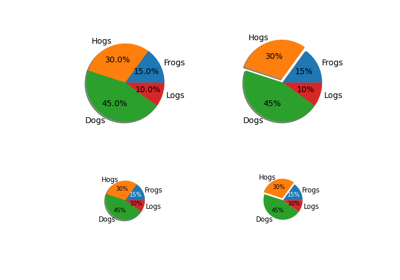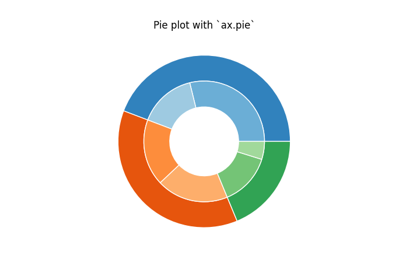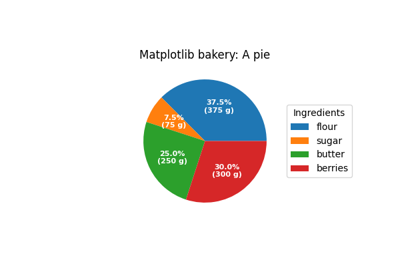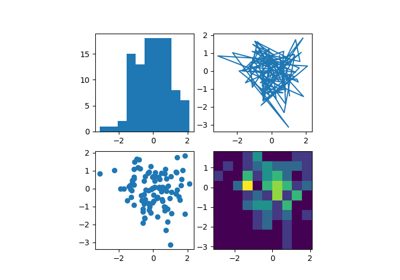# matplotlib.pyplot.pie¶

matplotlib.pyplot.pie(x, explode=None, labels=None, colors=None, autopct=None, pctdistance=0.6, shadow=False, labeldistance=1.1, startangle=None, radius=None, counterclock=True, wedgeprops=None, textprops=None, center=0, 0, frame=False, rotatelabels=False, *, data=None)[source]

Plot a pie chart.

Make a pie chart of array x. The fractional area of each wedge is given by x/sum(x). If sum(x) < 1, then the values of x give the fractional area directly and the array will not be normalized. The resulting pie will have an empty wedge of size 1 - sum(x).

The wedges are plotted counterclockwise, by default starting from the x-axis.

Parameters: xarray-likeThe wedge sizes. explodearray-like, optional, default: NoneIf not None, is a len(x) array which specifies the fraction of the radius with which to offset each wedge. labelslist, optional, default: NoneA sequence of strings providing the labels for each wedge colorsarray-like, optional, default: NoneA sequence of matplotlib color args through which the pie chart will cycle. If None, will use the colors in the currently active cycle. autopctNone (default), str, or function, optionalIf not None, is a string or function used to label the wedges with their numeric value. The label will be placed inside the wedge. If it is a format string, the label will be fmt%pct. If it is a function, it will be called. pctdistancefloat, optional, default: 0.6The ratio between the center of each pie slice and the start of the text generated by autopct. Ignored if autopct is None. shadowbool, optional, default: FalseDraw a shadow beneath the pie. labeldistancefloat or None, optional, default: 1.1The radial distance at which the pie labels are drawn. If set to None, label are not drawn, but are stored for use in legend() startanglefloat, optional, default: NoneIf not None, rotates the start of the pie chart by angle degrees counterclockwise from the x-axis. radiusfloat, optional, default: NoneThe radius of the pie, if radius is None it will be set to 1. counterclockbool, optional, default: TrueSpecify fractions direction, clockwise or counterclockwise. wedgepropsdict, optional, default: NoneDict of arguments passed to the wedge objects making the pie. For example, you can pass in wedgeprops = {'linewidth': 3} to set the width of the wedge border lines equal to 3. For more details, look at the doc/arguments of the wedge object. By default clip_on=False. textpropsdict, optional, default: NoneDict of arguments to pass to the text objects. centerlist of float, optional, default: (0, 0)Center position of the chart. Takes value (0, 0) or is a sequence of 2 scalars. framebool, optional, default: FalsePlot axes frame with the chart if true. rotatelabelsbool, optional, default: FalseRotate each label to the angle of the corresponding slice if true. patcheslistA sequence of matplotlib.patches.Wedge instances textslistA list of the label matplotlib.text.Text instances. autotextslistA list of Text instances for the numeric labels. This will only be returned if the parameter autopct is not None.

Notes

The pie chart will probably look best if the figure and axes are square, or the Axes aspect is equal. This method sets the aspect ratio of the axis to "equal". The axes aspect ratio can be controlled with Axes.set_aspect.

Note

In addition to the above described arguments, this function can take a data keyword argument. If such a data argument is given, the following arguments are replaced by data[<arg>]:

• All arguments with the following names: 'colors', 'explode', 'labels', 'x'.

Objects passed as data must support item access (data[<arg>]) and membership test (<arg> in data).

## Examples using matplotlib.pyplot.pie¶